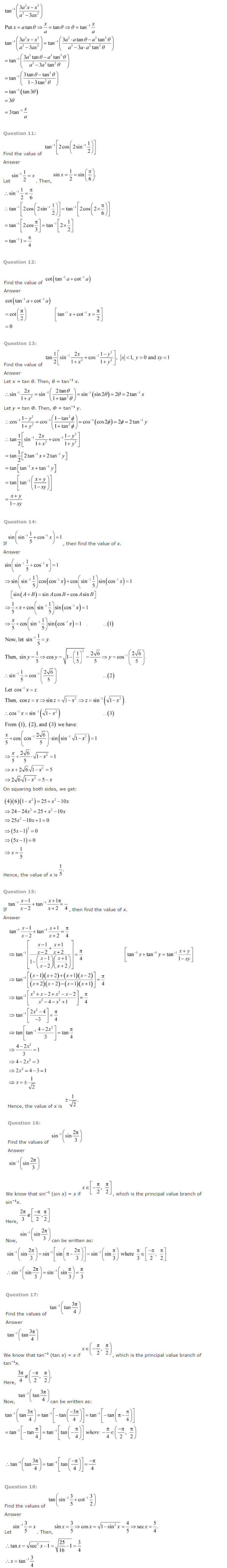# NCERT Solutions For Class 12 Maths Chapter 2 Inverse Trigonometric Functions

ncert textbook

## NCERT Solutions For Class 12 Maths Chapter 2 Inverse Trigonometric Functions

Topics and Sub Topics in Class 11 Maths Chapter 2 Inverse Trigonometric Functions:

 Section Name Topic Name 2 Inverse Trigonometric Functions 2.1 Introduction 2.2 Basic Concepts 2.3 Properties of Inverse Trigonometric Functions

NCERT Solutions for Class 12 Maths – Chapter 2 – Inverse Trigonometric Functions – is prepared by some of India’s best teachers. All the important topics are covered, each with a detailed explanation to help students understand the basic concepts better. NCERT books play a crucial role in the preparation for all exams conducted by the CBSE, including the JEE.

Chapter 2 – Inverse Trigonometric Functions covers multiple exercises. Answers to each question in every exercise are provided along with a step-by-step solution for better understanding by the student. This will prove to be most helpful to students in their home assignments as well as their practice sessions. The concepts included in the Inverse Trigonometric Functions chapter are the following: